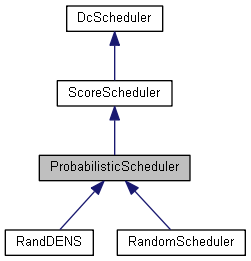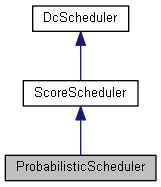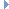GreenCloud Simulator
ProbabilisticScheduler Class Referenceabstract

`#include <probabilisticscheduler.h>`

Inheritance diagram for ProbabilisticScheduler:[legend]
Collaboration diagram for ProbabilisticScheduler:[legend]

## Public Member Functions

ProbabilisticScheduler ()

virtual ~ProbabilisticScheduler ()Public Member Functions inherited from ScoreScheduler
ScoreScheduler ()

virtual ~ScoreScheduler ()Public Member Functions inherited from DcScheduler
DcScheduler ()

virtual ~DcScheduler ()

## Private Member Functions

virtual double calculateScore (ResourceProvider *rp)=0

## Detailed Description

Definition at line 15 of file probabilisticscheduler.h.

## Constructor & Destructor Documentation

 ProbabilisticScheduler::ProbabilisticScheduler ( )

Definition at line 10 of file probabilisticscheduler.cc.

10  {
11
12
13 }
 ProbabilisticScheduler::~ProbabilisticScheduler ( )
virtual

Definition at line 15 of file probabilisticscheduler.cc.

15  {
16
17 }

## Member Function Documentation

 virtual double ProbabilisticScheduler::calculateScore ( ResourceProvider * rp )
privatepure virtual

Implements ScoreScheduler.

Implemented in RandDENS, and RandomScheduler.

virtual

Implements DcScheduler.

Reimplemented in RandDENS, and RandomScheduler.

Definition at line 19 of file probabilisticscheduler.cc.

19  {
20  //1. calculate mDENS score array
21  //2. generate random number in [0:summed mDENS score]
22  //3. binary search on the array
23  //4. return the selected host
24  TskComAgent* selected = NULL;
25  std::vector<double> mdens_score(providers.size());
26  std::vector<double> mdens_score_cumulative(providers.size());
27  vector <ResourceProvider*>::iterator res_p;
28  vector <double>::iterator score;
29  vector <double>::iterator cumul;
30  for (res_p = providers.begin(), score = mdens_score.begin(), cumul = mdens_score_cumulative.begin();
31  res_p!=providers.end();
32  res_p++, score++, cumul++)
33  {
34  (*score) = calculateScore((*res_p));
35  if(cumul==mdens_score_cumulative.begin()){
36  (*cumul) = (*score);
37  } else {
38  (*cumul) = (*score) + (*(cumul-1));
39  }
40 // std::cout << "score:" << (*score)<< " cumul: " << (*cumul) << "\n";
41  }
42 // srand(time(0));
43  double r = ( (double)rand() / (double)RAND_MAX ) * mdens_score_cumulative.at(mdens_score_cumulative.size()-1);
44 // std::cerr << "Random: " << r << "\n";
45  vector <double>::iterator lb = lower_bound(mdens_score_cumulative.begin(),mdens_score_cumulative.end(),r);
46  int sel_ind = lb - mdens_score_cumulative.begin();
47 // std::cerr << "Lb: "<< (*lb) << " ind: " << sel_ind << "\n";
48 // abort();
49  selected = providers.at(sel_ind)->getTskComAgent();
50  return selected;
51 }
virtual double calculateScore(ResourceProvider *rp)=0

The documentation for this class was generated from the following files: